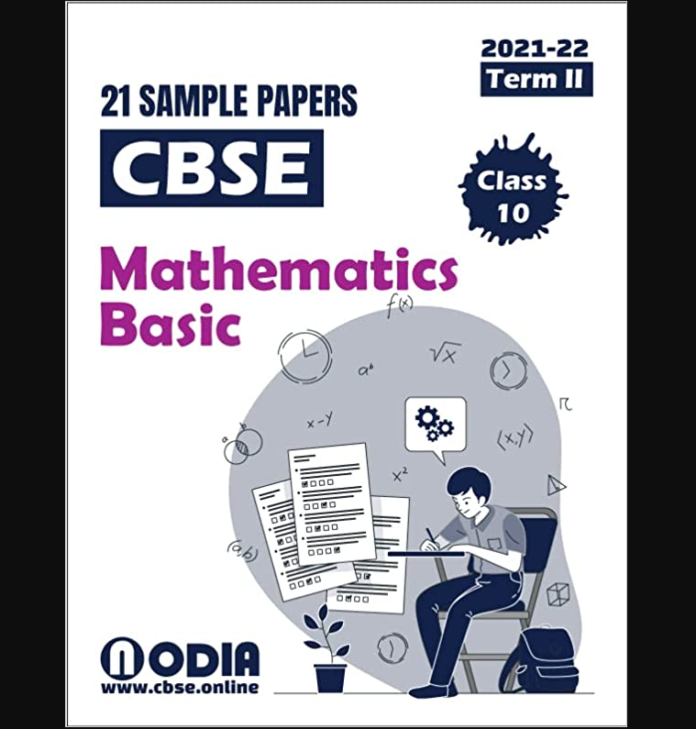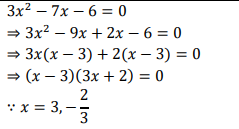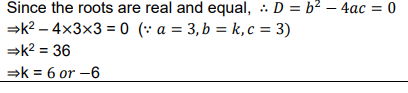# Sample Question Paper Maths- Basic (241) Class 10, Session: 2021-22 TERM 2

CBSE class 10th Mathematics- Basic sample question paper## Ques 1- Find the roots of the quadratic equation 3𝑥2 − 7𝑥 − 6 = 0.

### Solution :OR

## Find the values of k for which the quadratic equation 3𝑥2 + 𝑘𝑥 + 3 = 0 has real and equal roots.

### Solution :